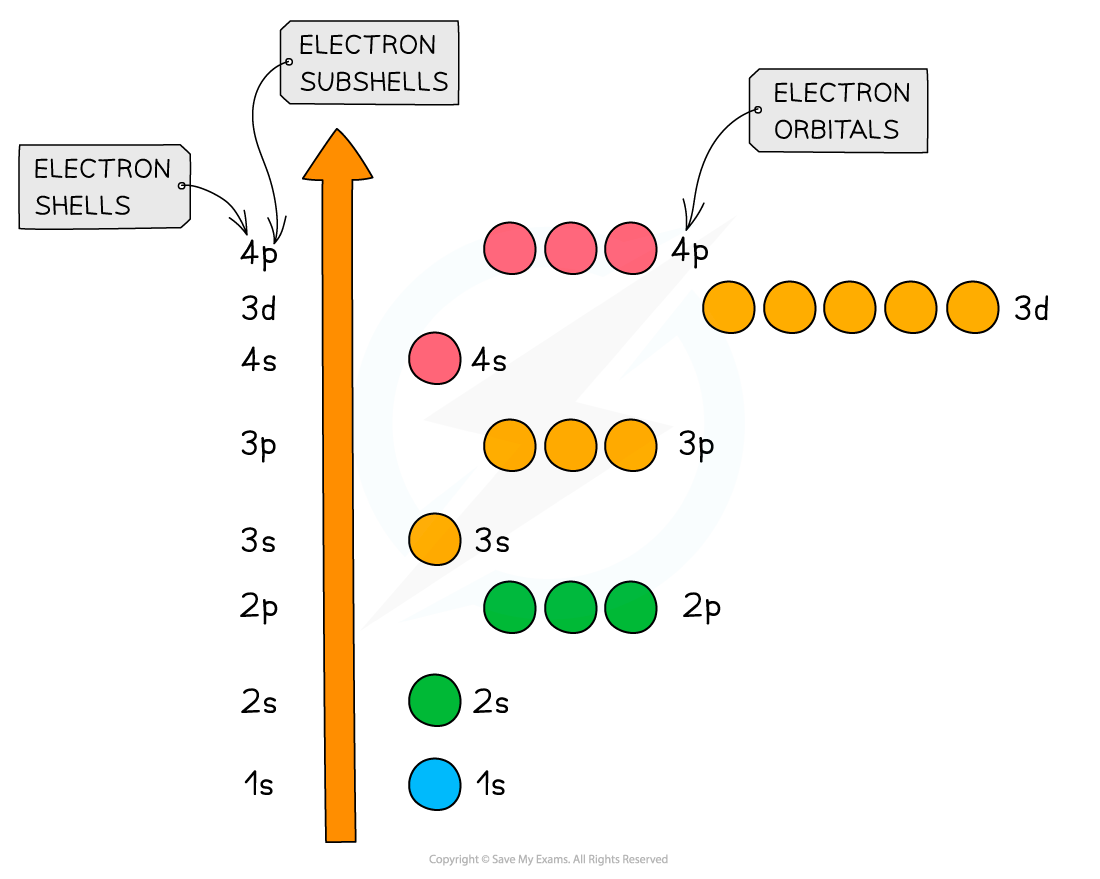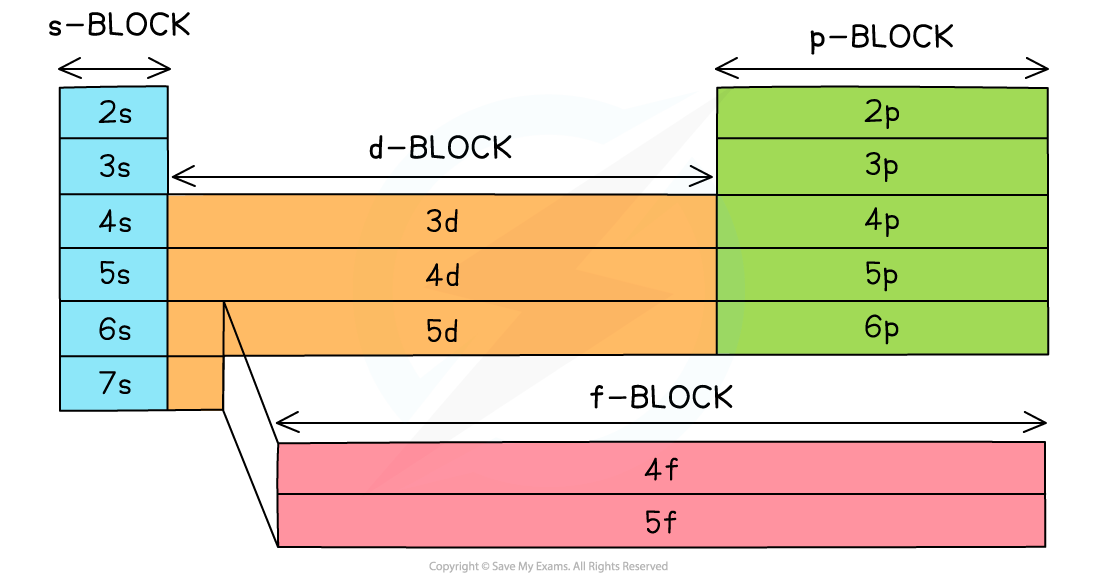# Edexcel A Level Chemistry:复习笔记1.2.4 Electronic Configurations

### Electronic Configurations of Atoms & Ions

• The principal quantum shells increase in energy with increasing principal quantum number
• E.g. n = 4 is higher in energy than n = 2

• The subshells increase in energy as follows: s ＜ p ＜ d ＜ f
• The only exception to these rules is the 3d orbital which has slightly higher energy than the 4s orbital
• Because of this, the 4s orbital is filled before the 3d orbital

• All the orbitals in the same subshell have the same energy and are said to be degenerate
• E.g. px, py and pz are all equal in energyRelative energies of the shells and subshells

• The electron configuration gives information about the number of electrons in each shell, subshell and orbital of an atom
• The subshells are filled in order of increasing energyThe electron configuration shows the number of electrons occupying a subshell in a specific shell

• Writing out the electron configuration tells us how the electrons in an atom or ion are arranged in their shells, subshells and orbitals
• This can be done using the full electron configuration or the shorthand version
• The full electron configuration describes the arrangement of all electrons from the 1s subshell up
• The shorthand electron configuration includes using the symbol of the nearest preceding noble gas to account for however many electrons are in that noble gas

• Ions are formed when atoms lose or gain electrons
• Negative ions are formed by adding electrons to the outer subshell
• Positive ions are formed by removing electrons from the outer subshell
• The transition metals fill the 4s subshell before the 3d subshell but lose electrons from the 4s first and not from the 3d subshell (the 4s subshell is lower in energy

#### Full Electron Configurations

• Hydrogen has 1 single electron
• The electron is in the s orbital of the first shell
• Its electron configuration is 1s1

• Potassium has 19 electrons
• The first 2 electrons fill the s orbital of the first shell
• They then continue to fill subsequent orbitals and subshells in order of increasing energy
• The 4s orbital is lower in energy than the 3d subshell, so it is therefore filled first
• The full electron configuration of potassium is 1s2 2s2 2p6 3s2 3p6 4s1

#### Shorthand Electron Configurations

• Using potassium as an example again:
• The nearest preceding noble gas to potassium is argon
• This accounts for 18 electrons of the 19 electrons that potassium has
• The shorthand electron configuration of potassium is [Ar] 4s1

#### Worked Example

Write down the full and shorthand electron configuration of the following elements:

1.   Calcium
2.   Gallium
3.   Ca2+

• Calcium has 20 electrons so the full electronic configuration is:

1s2 2s2 2p6 3s2 3p6 4s2

• The 4s orbital is lower in energy than the 3d subshell and is therefore filled first
• The shorthand version is [Ar] 4s2 since argon is the nearest preceding noble gas to calcium which accounts for 18 electrons

• Gallium has 31 electrons so the full electronic configuration is:

1s2 2s2 2p6 3s2 3p6 3d10 4s2 4p1

[Ar] 3d10 4s2 4p1

• Even though the 4s is filled first, the full electron configuration is often written in numerical order. So, if there are electrons in the 3d sub-shell, then these will be written before the 4s

• What this means is that if you ionise calcium and remove two of its outer electrons, the electronic configuration of the Ca2+ ion is identical to that of argon

Ca2+ is 1s2 2s2 2p6 3s2 3p6

Ar is also 1s2 2s2 2p6 3s2 3p6

#### Exceptions

• Chromium and copper have the following electron configurations, which are different to what you may expect:
• Cr is [Ar] 3d5 4s1 not [Ar] 3d4 4s2
• Cu is [Ar] 3d10 4s1 not [Ar] 3d9 4s2

• This is because the [Ar] 3d5 4s1 and [Ar] 3d10 4s1 configurations are energetically stable

#### Presenting the Electron Configuration

• Electrons can be imagined as small spinning charges which rotate around their own axis in either a clockwise or anticlockwise direction
• The spin of the electron is represented by its direction

• Electrons with similar spin repel each other which is also called spin-pair repulsion
• Electrons will therefore occupy separate orbitals in the same subshell where possible, to minimize this repulsion and have their spin in the same direction
• E.g. if there are three electrons in a p subshell, one electron will go into each px, py and pz orbitalElectron configuration: three electrons in a p subshell

• Electrons are only paired when there are no more empty orbitals available within a subshell, in which case the spins are the opposite spins to minimize repulsion
• E.g. if there are four electrons in a p subshell, one p orbital contains 2 electrons with opposite spin and two orbitals contain one electron only
• The first 3 electrons fill up the empty p orbitals one at a time, and then the 4th one pairs up in the px orbitalElectron configuration: four electrons in a p subshell

#### Box Notation

• The electron configuration can be represented using the electrons in boxes notation
• Each box represents an atomic orbital
• The boxes are arranged in order of increasing energy from bottom to top
• The electrons are represented by opposite arrows to show the spin of the electrons
• E.g. the box notation for titanium is shown below
• Note that since the 3d subshell cannot be either full or half full, the second 4s electron is not promoted to the 3d level and stays in the 4s orbitalThe electrons in titanium are arranged in their orbitals as shown. Electrons occupy the lowest energy levels first before filling those with higher energy

### Classifying Elements

• The Periodic Table is split up into four main blocks depending on their electron configuration
• Elements can be classified as an s-block element, p-block element and so on, based on the position of the outermost electron:
• s block elements - Have their valence electron(s) in an s orbital
• p block elements - Have their valence electron(s) in a p orbital
• d block elements - Have their valence electron(s) in a d orbital
• f block elements - Have their valence electron(s) in an f orbital### Electronic Configuration & Chemical Properties

• The way an element reacts with other elements is determined by an number of factors, but in particular the electronic configuration of its atoms
• The Periodic Table is arranged in periods (horizontal) and groups (vertical)
• All the elements in the same period have the same number of shells (principle quantum number)
• All the elements in the same group have the same number of outer electrons
• The elements in each group of period show particular trends and characteristics in their chemical and physical properties that can be explained in terms of their atomic numbers
• This provides valuable information about what is likely to happen when particular elements react
• The properties of the elements are a function of their atomic numbers
• Using this information as well as the location of the elements in the different blocks we can predict the properties
• For example:
• Helium, neon and argon all have electronic structures with full sub shells as well as high ionisation energies
• This confirms that the electronic arrangement is very stable explaining why they rarely react with other chemicals